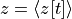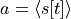# ANN Statistics Sampler

ANN sampler module.

class lava.lib.dl.bootstrap.ann_sampler.AnnSampler(num_centers=5, sample_range=[0.1, 0.9], eps=1e-05)

ANN data point sampler. It samples the weighted spike input rateand neurons output spike rate (activation)data points, manages the data points, and provides picewise linear ANN activation.

Parameters
• num_centers (int) – number of sampling centers. Defaults to 5.

• sample_range (list) – min and max range of sampling points. Defaults to [0.1, 0.9].

• eps (float) – infinitesimal constant. Defaults to 1e-5.

append(a, z)

Appends new data points

Parameters
• a (torch.tensor) – output spike tensor.

• z (torch.tensor) – weighted spike tensor.

clear()

Clears all data points.

fit()

Fit piecewise linear model from sampled data.

forward(z)

Picewise ANN activation

Parameters

z (torch tensor) – weighted input for ANN.

Returns

equivalent ANN activation.

Return type

torch tensor

plot(figsize=None)

Plots the piecewise ANN activation.

Parameters

figsize (tuple of 2) – width and height of figure. Defaults to None.

soft_clear()

Randomly clears half of the data points while retaining some of the history.